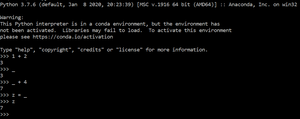Skip to content
Related Articles
Role of Underscores ‘_’ in Python
• Last Updated : 01 Oct, 2020

The Underscore (_) is an eccentric character in Python. It can be used in many ways in a Python program.

### The various uses of underscore (_) in Python are:

1) Use in Interpreter:

Python immediately saves the value of the last expression in the interpreter in this unique variable. Underscore (_) can also be used to value of any other variable.

Example 1:Underscore (_) can be also be used as a normal variable.

Example 2:

## Python3

 `# Storing value in _``_ ``=` `2` `+` `8`` ` `print``(_)`

Output:

```10
```

2) Use in Loops:

In Python underscore (_) can be used as a variable in looping. It will access each element of the data structure.

Example 1:

## Python3

 `# Creating tuple``Tuple` `=` `(``50``, ``40``, ``30``)`` ` `# Using _ to access index of each element``for` `_ ``in` `range``(``3``):``    ``print``(``Tuple``[_])`

Output:

```50
40
30
```

Example 2:

## Python3

 `# Creting list``List` `=` `[``'Geeks'``, ``4``, ``'Geeks!'``]`` ` `# Using _ to access elements of list``for` `_ ``in` `List``:``    ``print``(_)`

Output:

```Geeks
4
Geeks!
```

3) Use in Ignoring Variables:

In Python underscore (_) is often used to ignore a value. If one doesn’t use some values when unpacking, just set the value to underscore (_). Ignoring involves assigning values to a particular vector underscore (_). We add values to underscore (_) if this is not used in future code.

Example 1:

## Python3

 `# Using _ to ignore values``p, _, r ``=` `'Geeks'``, ``4``, ``'Geeks!'`` ` `print``(p, r)`

Output:

```Geeks Geeks!
```

Example 2:

## Python3

 `# Using _ to ignore multiple values``p, q, ``*``_, r ``=` `'Geeks'``, ``4``, ``'randomText'``, ``1234``, ``'3.14'``, ``"Geeks!"`` ` `print``(p, q, r)`` ` `print``(_)`

Output:

```Geeks 4 Geeks!
['randomText', 1234, '3.14']
```

4) Separating digit of Numbers:

Underscores (_) can also be used to represent long digits number, it separates the group of digits for better understanding.

## Python3

 `# Using _ to seperate digits``Crore ``=` `10_00_000`` ` `print``(Crore)`

Output:

```1000000
```

5) Use in Defining Access of Data members and Methods in Class:

Underscore (_) is used as a prefix for a method or data member in a class, defines its Access Specifier, and using double underscores (__) as both suffix and prefix refer to a Constructor.

Example 1:

## Python3

 `class` `Gfg:``    ``a ``=` `None``    ``_b ``=` `None``    ``__c ``=` `None``     ` `    ``# Constructor``    ``def` `__init__(``self``, a, b, c):`` ` `        ``# Data mambers``        ``# Public``        ``self``.a ``=` `a``         ` `        ``# Protected``        ``self``._b ``=` `b``         ` `        ``# Private``        ``self``.__c ``=` `c`` ` `    ``# Methods``    ``# Private method``    ``def` `__display(``self``):``        ``print``(``self``.a)``        ``print``(``self``._b)``        ``print``(``self``.__c)``    ``# Public method``    ``def` `accessPrivateMethod(``self``):``        ``self``.__display()`` ` ` ` `# Driver code``# Creating object``Obj ``=` `Gfg(``'Geeks'``, ``4``, ``"Geeks!"``)`` ` `# Calling method``Obj.accessPrivateMethod()`

Output:

```Geeks
4
Geeks!
```

Attention geek! Strengthen your foundations with the Python Programming Foundation Course and learn the basics.

To begin with, your interview preparations Enhance your Data Structures concepts with the Python DS Course. And to begin with your Machine Learning Journey, join the Machine Learning – Basic Level Course

My Personal Notes arrow_drop_up